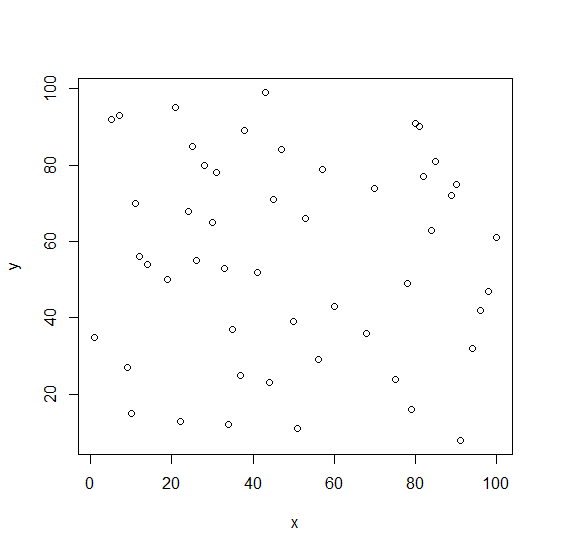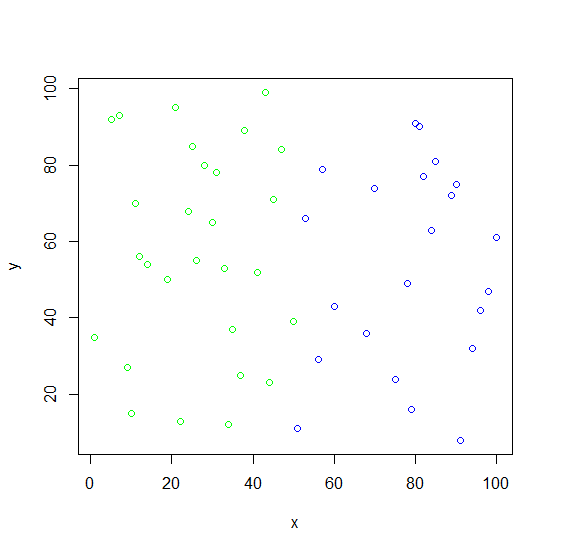# How to create a scatterplot with colors as group in R?

To create a scatterplot with colors as group, we can define the colors in col argument as characters.

For Example, if we have two vectors then we can create scatterplot between x and y with red and blue colors for x and y value by using the below mentioned command −

plot(x,y,col=as.character(cut(x,2,labels=c("red","blue"))))

## Example

Following snippet creates a sample data frame −

x<-sample(1:100,50)
x

The following dataframe is created

  51 85 47 78 96 9 5 94 12 53 81 10 14 34 91 75 35 31 90
 24 41 68 19 28 7 22 100 45 70 98 30 11 56 25 82 26 37 33
 84 50 80 43 1 60 89 38 79 57 44 21

Following snippet creates a sample data frame −

y<-sample(1:100,50)
y

The following dataframe is created

  11 81 84 49 42 27 92 32 56 66 90 15 54 12 8 24 37 78 75 68 52 36 50 80 93
 13 61 71 74 47 65 70 29 85 77 55 25 53 63 39 91 99 35 43 72 89 16 79 23 95

To create a scatterplot with colors as group on the above created data frame, add the following code to the above snippet −

x<-sample(1:100,50)
y<-sample(1:100,50)
plot(x,y)

## Output

If you execute all the above given snippets as a single program, it generates the following Output −To create a scatterplot with colors as group on the above created data frame, add the following code to the above snippet −

x<-sample(1:100,50)
y<-sample(1:100,50)
plot(x,y,col=as.character(cut(x,2,labels=c("green","blue"))))

## Output

If you execute all the above given snippets as a single program, it generates the following Output −